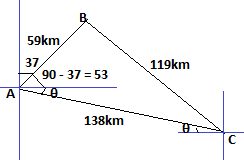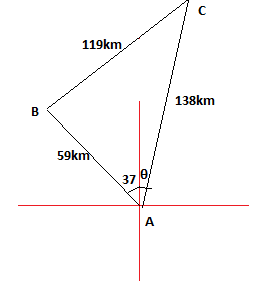mycollegehive
What is the bearing of C from A
Math0A girl travels from garage A for 59km to garage B on a bearing of 037degree  and then turns right through 90degree and travels for 119km to garage C. What is the bearing of C from A if /AC/=138km

49 viewsShareFollowNigeria
13 September 2020School not set Nigeria
14 September 20200x = 53 + θ ---------- eqn(1)

opp = 119

tanx = 119/59

tanx = 2.0169

x = tan-1(2.0169)

x = 63.63

from eqn(1),

θ = x - 53

= 63.63 - 53

= 10.63

Thus, the bearing from C to A = 90 + 10.63 = 100.63ShareSchool not set Nigeria
14 September 20200ABC is a triangle.

From pythagoras theorem, sinx = opp/hyp

x = θ + 37

opp = 119

hyp = 138

sin(θ + 37) = 119/138

sin(θ + 37) = 0.8623

θ + 37 = sin-1(0.8623)

θ + 37 = 59.58

θ = 59.58 - 37

θ = 22.58

OR

θ = 22.58 + 180 = 202.58

Therefore, the bearing from C to A is 22.58 or 202.58Share### Related Tags

Math

9 followers

1018 questions